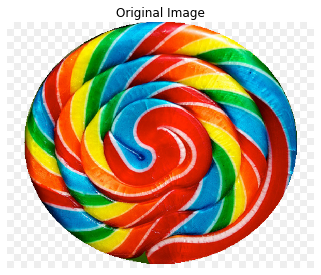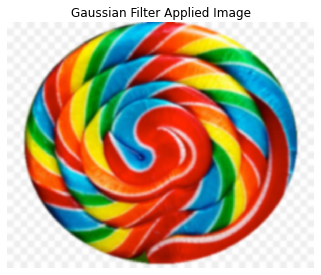# Image Processing Tutorial Using scikit-image - Noise

Betul Mescioglu

## Smoothing Edges in an Image: Gaussian Filter

Applying a Gaussian filter reduces the image's high frequency components. Thus, it is called a low pass filter. It is often used to remove Gaussian (random) noise from the image. Skimage.filters module has a function called gaussian() that employs this feature on images. It takes a parameter called sigma that determines how much of the noise will be removed. Larger sigma values may remove more noise, but they will also remove more details from the image.

``````#Importing necessary libraries
import skimage.io
import matplotlib.pyplot as plt
import skimage.filters
from skimage.util import random_noise
from skimage.restoration import denoise_tv_chambolle, denoise_bilateral

#Displaying the image
skimage.io.imshow(candy)
plt.title('Original Image')
plt.axis('off')``````
`(-0.5, 899.5, 719.5, -0.5)`Let's apply a Gaussian filter to the candy image:

``````gauss_blurred = skimage.filters.gaussian(candy, sigma=(3,3), truncate=3.5,multichannel=True)
skimage.io.imshow(gauss_blurred)
plt.title('Gaussian Filter Applied Image')
plt.axis('off')``````
`(-0.5, 899.5, 719.5, -0.5)`We observe that the sharpness of the image is reduced and colors blend in more.

## Adding Noise to an Image:

We can add noise to an image using skimage. Here, we will add random noise to a landscape image using Gaussian, Salt & Pepper and Poisson distributions.

``````#Adding noise to an image:
g_noised_image = random_noise(land, mode='gaussian')
sp_noised_image = random_noise(land, mode='s&p', amount=0.09)
poisson_noised_image =random_noise(land, mode='poisson')
#Plot all noised images with the original image:
fig, ax = plt.subplots(nrows=2,ncols=2, figsize=(12,6), sharex=True, sharey=True, squeeze=True)
ax = ax.ravel()
ax.imshow(land)
ax.set_title('Original Image')
ax.imshow(g_noised_image)